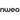# How to divide fractions: 3 easy steps to solve hard problems

Ryan Juraschka

All Posts
Teaching your students how to divide fractions can be just as simple as teaching multiplication... once you know all of the little tricks to get the right answer. But -- as with any math concept -- when you teach division, you don't want your students to just solve a problem. You want them to understand what's happening in each question. But, that's the thing. It's tough to get them to understand dividing fractions if you don't quite get it yourself. We were a bit confused on the subject, too. That's why we looked into the best tools and easiest ways to make sure your class understands the key concepts for dividing fractions. Pay close attention, and by the end of this article you’ll be a fully equipped, ultra confident fraction division master.

## How dividing fractions works

Teaching students how to divide fractions is part of the Common Core State Standards for Mathematical Practice. One of the most valuable things to teach your students when dividing fractions is what the answer means. Take a look at the example below:

½ ÷ ⅙ = 3

Why is the solution a bigger number than the fractions involved?When you divide a fraction, you’re asking how many groups of the divisor (second number) can be found in the dividend (first number). For the above equation, we’re asking how many ⅙ appear in ½. Imagine the example equation as a cake. You’ve got half of the cake remaining. If each serving of the cake is ⅙ of the whole, how many servings do you have left?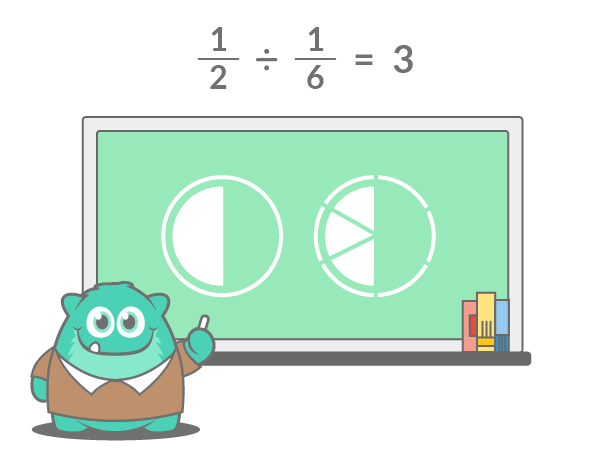As you can see, you’ve got three servings of cake remaining!

## How to divide fractions

If you simply divided fractions like you were dividing a normal math problem, you’d more than likely create some complex fractions and have something that looks similar to this:   [caption id="attachment_3424" align="aligncenter" width="388"]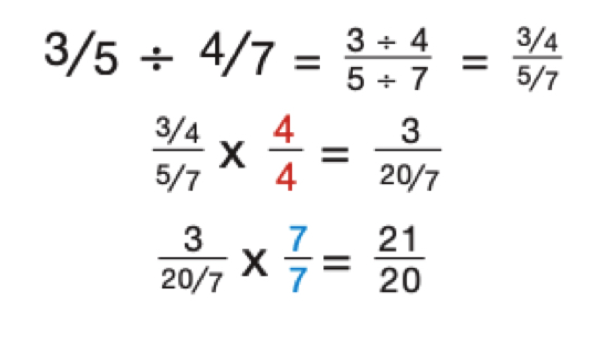Credit: Mike's Math Club[/caption] That’s not exactly an easy process to follow.Luckily, there’s a shortcut you can use that makes dividing fractions much simpler. You can solve most division problems by following these three steps:
1. Flip the divisor into a reciprocal
2. Change the division sign to a multiplication symbol and multiplyThese steps streamline the dividing process and help you avoid complex fractions.

### Step 1: Flip the divisor into a reciprocal

A reciprocal is what you multiply a number by to get the value of one. If you want to change two into one through multiplication you need to multiply it by 0.5. In fraction form this looks like:

²⁄₁ × ½ = 1

To find the reciprocal of a fraction you simply flip the numbers. The denominator becomes the numerator and vice versa.Take a look at the example equation again:

½ ÷ ⅙ = ?

The first step to solve the problem is to turn our divisor, ⅙, into a reciprocal.

⅙ → ⁶⁄₁

### Step 2: Change the division sign to a multiplication symbol and multiply

Dividing and multiplying are opposites of each other. When you create a reciprocal of a number, you’re creating its opposite as well. In a division problem, when you turn the divisor into a reciprocal, you also need to change the equation from division to multiplication.Now that you’ve found the reciprocal of your divisor, you can change the equation from division into multiplication.

½ ÷ ⅙ = ? → ½ × ⁶⁄₁ = ?

We’ve got an extensive guide on how to multiply fractions, but here’s a quick tutorial:
3. Simplify the final fraction, if possible
For the example equation you have two problems to solve:

1 × 6 = 6     2 × 1 = 2    ½ × ⁶⁄₁ = ⁶⁄₂

Fractions symbolize a part of a whole. This means many fractions represent the same value, so why not make the fraction as simple as possible?For example, you almost never say five-tenths or ⁵⁄₁₀. Instead, you simplify that to one-half or ½.To get a fraction down to its simplest form, you divide the numerator and denominator by their greatest common factor. The greatest common factor in ⁵⁄₁₀ is five. Dividing both numbers down by five leaves you with ½.In the example question, the greatest common factor of ⁶⁄₂ is two. This turns your solution from ⁶⁄₂ to ³⁄₁, which is the same as saying three.Therefore:

½ ÷ ⅙ = ? → ½ × ⁶⁄₁ = ⁶⁄₂ → ³⁄₁ → 3

Creating a reciprocal and multiplying an equation rather than dividing lets you skip several steps in an equation. It’s a shortcut that will make your students’ lives a whole lot easier!

## Dividing fractions examples

The three-step strategy is great for basic fraction problems, but what happens when you run into whole numbers, mixed fractions, improper fractions, and word-based problems?The process remains the same for the most part, but depending on the type of problem, there could be a couple more steps.Let’s look at some examples of different types of problems:

### How to divide improper fractions

[caption id="attachment_3342" align="aligncenter" width="395"]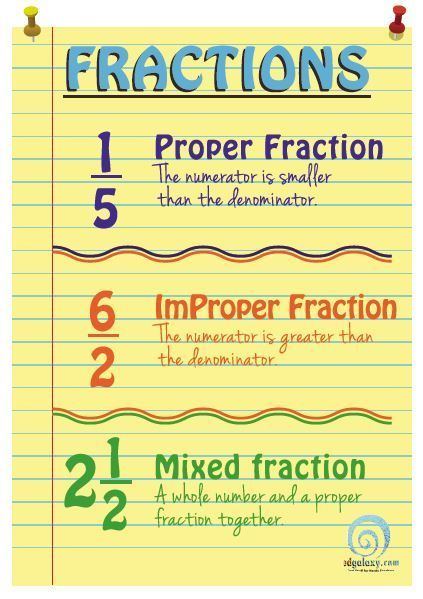Credit: edgalaxy[/caption] An improper fraction is when you have a numerator with a value that is greater than the denominator. Seeing these fractions can cause confusion, but the order of operations does not change.Example 1:

⅓ ÷ ⁶⁄₅ = ? → ⅓ × ⅚ = ⁵⁄₁₈

Example 2:

⁷⁄₆ ÷ ¾ = ? →⁷⁄₆ × ⁴⁄₃ = ²⁸⁄₁₈ → ¹⁴⁄₉ → 1 ⁵⁄₉

No matter where the improper fraction is placed, you still flip the divisor into a reciprocal and then multiply the two fractions.

### How to divide mixed fractions

[caption id="attachment_3353" align="aligncenter" width="600"]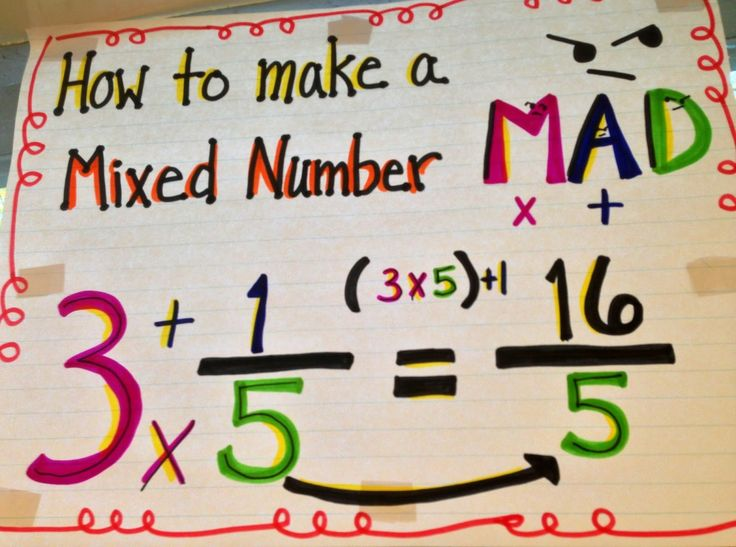Credit: Fabulous Finch Facts[/caption] A mixed fraction is when you have a whole number along with a fraction. For example, 2 ½ would be considered a mixed fraction. How do you divide a mixed fraction?Change your mixed fraction into an improper fraction and then proceed with the three-step strategy. To do this, multiply your whole number by the denominator. Then take that value and add it to your numerator.2 ½ changes to ⁵⁄₂.Example 1:

3 ⅓ ÷ ⅖ = ? → ¹⁰⁄₃ ÷ ⅖ = ? → ¹⁰⁄₃ × ⁵⁄₂ = ⁵⁰⁄₆ → ²⁵⁄₃ → 8 ⅓

Example 2:

¼ ÷ 2 ⅙ = ? → ¼ ÷ ¹³⁄₆ = ? → ¼ × ⁶⁄₁₃ = ⁶⁄₅₂ → ³⁄₂₆

Example 3:

2 ½ ÷ 1 ⅓  = ? → ⁵⁄₂ ÷ ⁴⁄₃= ? → ⁵⁄₂ × ¾ = ¹⁵⁄₈ → 1 ⅞

### How to divide fractions with whole numbers

[caption id="attachment_3357" align="aligncenter" width="600"]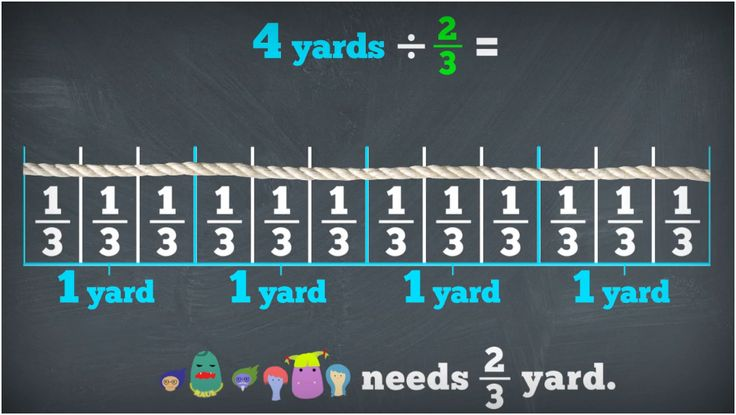Credit: PBS LearningMedia[/caption] Questions with whole numbers are similar to problems with mixed fractions. Before you can start dividing, you need to turn the whole number into a fraction.To turn a whole number into a fraction, make the numerator the whole number and make the denominator one.

3 → ³⁄₁

Once the whole number is turned into a fraction you can continue to solve the problem with the three-step strategy.Example:

⅓ ÷ 3 = ? → ⅓ ÷ ³⁄₁= ? → ⅓ × ⅓ = ⅑

### How to divide fractions with the same denominator

When you’ve got the same denominator, there’s no need to find the reciprocal or multiply. You can simply divide your fractions to get the answer. The denominators will cancel each other out and give you one.Any fraction with a denominator of one can be simplified to just the numerator.Example 1:

⅘ ÷ ⅖ = ²⁄₁ → 2

Example 2:

⅓ ÷ ⅔ = ½/1 → ½

### Dividing fractions word problemsWord problems can be tricky because you’ve got to teach your students to understand which value becomes the dividend and which will become the divisor.Like all division problems, in a word problem you’re trying to find out how many groups of one number can be found in another. The best way to understand which number is which is by looking at an example.There is a 25 ½ kilometer stretch of highway that needs to be repaired. The construction crew for the job can repair 4 ¼ kilometers of road per week. How many weeks will it take to repair the highway? In this equation, you’re looking for the number of weeks it takes to repair a highway. To get that answer, you need to see how many groups of 4 ¼ (the amount of highway that can be repaired per week) can fit into 25 ½ (the total length of highway that needs to be repaired). Therefore, 25 ½ will be your dividend and 4 ¼ will be your divisor!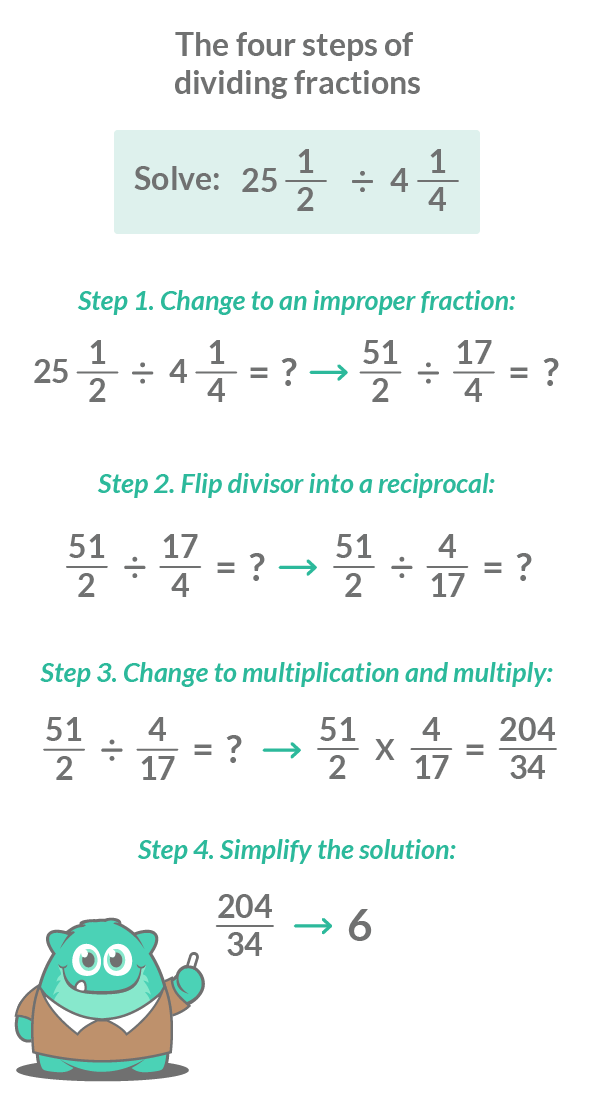After plugging the numbers into the correct places you’ll find it will take six weeks to repair the highway.To make sure your students are following along, you can work through word problems together and then ask them to raise their hand if they think one number is the dividend. Then, ask again to see if they think the other number is the dividend.Next, pick a student to explain why one number is the dividend and why the other is the divisor. This will not only get students involved but it’ll also give you a chance to see how students are processing the material you’re teaching!

Prodigy Game helps you teach how to divide fractions, track how your students are doing, and assign specific questions to prepare your class for standardized testing – all for free.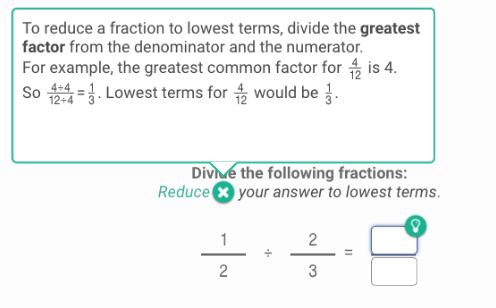The math game gets your students excited to learn – and most of the time they don’t even realize that they’re being tested. You have several options, including the ability to focus in-game questions around topics that you are teaching, up-to-date statistics, and progress reports.Here's how you can use Prodigy in your classroom to: Prodigy works faster than worksheets as all of the “marking” is done in for you – and in real time. You can look at reports on your whole class and see which topics different students are struggling with!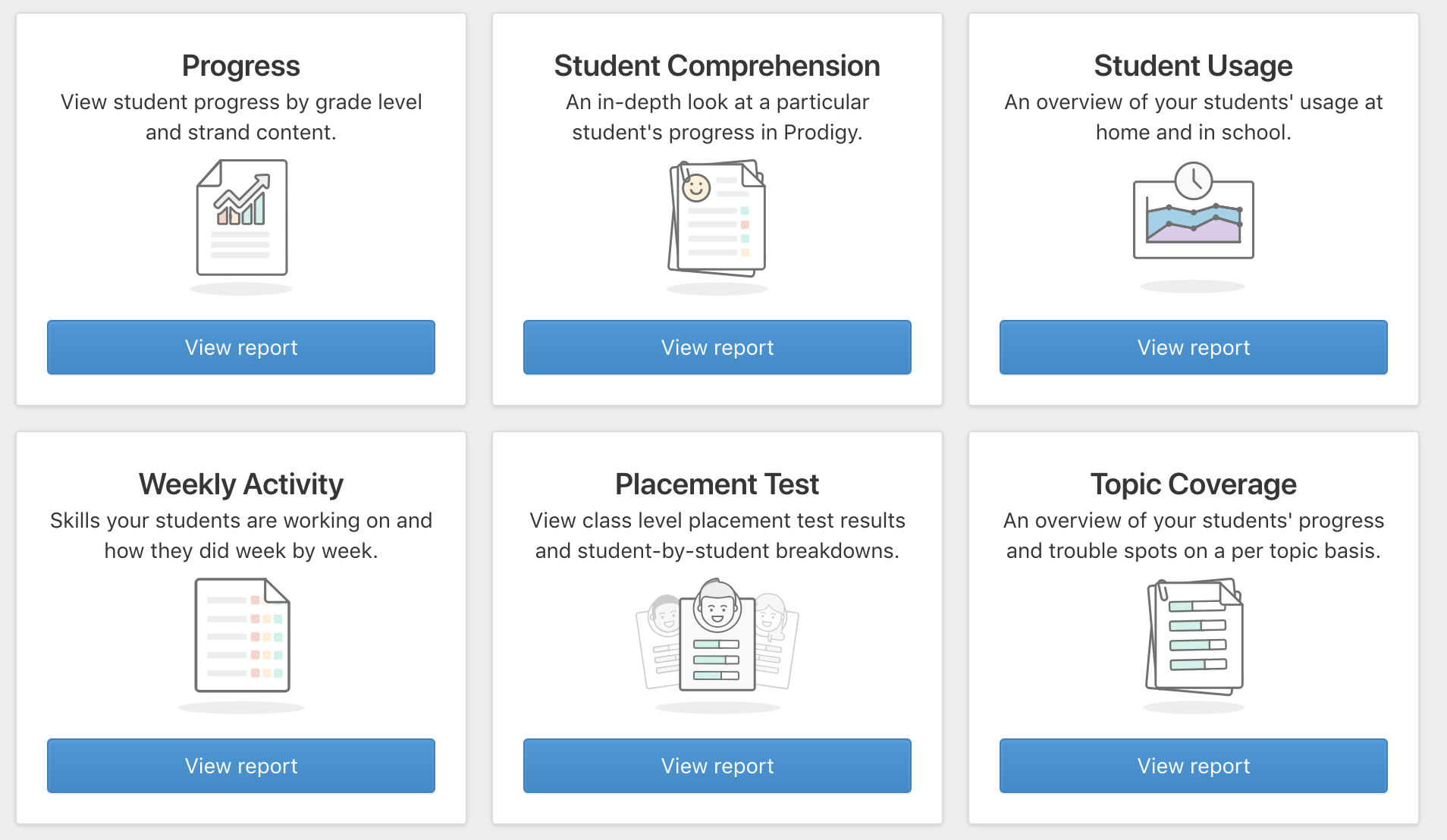You can also create assignments for each student based on their specific needs and learning styles. All of your students will be given the chance to practice questions they have trouble with and improve their overall math skills.When test time is around the corner, you can create a practice test in-game to see if any topics need to be covered in class with more depth.Prodigy Game is always free for teachers.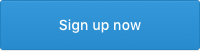## Dividing fractions worksheets

To make sure key concepts are getting across when teaching how to divide fractions you can also use worksheets for your class. You can put an assortment of different questions in a worksheet to see what students understand and what they're struggling with.The only drawback with worksheets is that they can take a long time to mark. The longer it takes to mark, the longer it will take to see what your students need help with. Here are some websites to get worksheets that you can try in your classroom: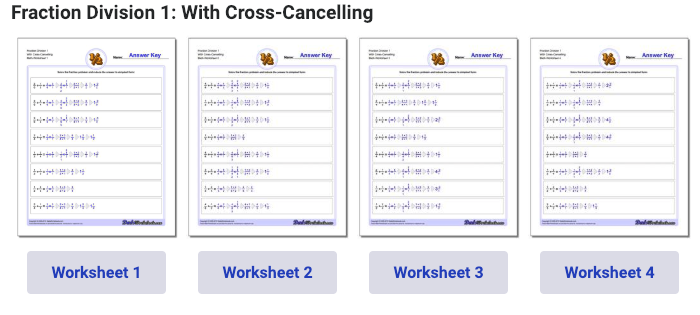DadsWorksheets.com gives you a wide variety of worksheets to choose from depending on the topic you’re working on. All worksheets come with an answer key to make things easier. Everything they offer is downloadable and printable right from the website.

### 2. Common Core Sheets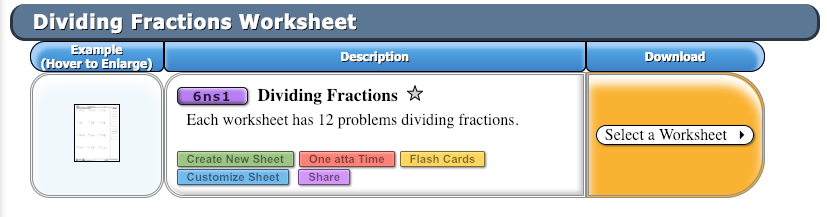Common Core Sheets takes your worksheets to the next level by allowing you to customize your lessons. You can select the types of questions that you want to appear on your worksheets. You also get to select if you want fractions simplified or turned into mixed numbers for answers.The final product gives you two worksheets. The first only has the questions, while the second has all of the answers, including the process to get the solution.

### 3. K5 LearningK5 learning provides worksheets for classes Kindergarten to the fifth grade. They cover many topics seen in a curriculum and each subject has several worksheets to use. The PDF that you can download from their website includes worksheets and the answer key.

## Dividing fractions calculator

When your students are learning how to divide fractions you can show them fraction calculators. These are online tools for solving fraction problems quickly. They’re great for checking your answers, but use caution when showing these tools to your class.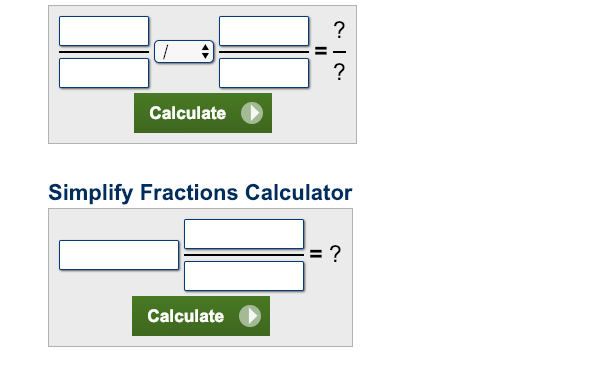Fraction calculators can be used when students are doing homework, but you don’t want them to rely on them to solve questions or else they won’t learn anything. If you use Calculator.net they show you all of the different steps you need to take to solve a problem!

## Final thoughts on how to divide fractions

When teaching how to divide fractions, tell your students that they're trying to find how many of the divisor can be found in the dividend. The easiest way to divide fractions is to follow three simple steps:
1. Flip the divisor into a reciprocal
This method creates a shortcut so that you don’t have to deal with complex fractions when solving a problem. Finding the reciprocal and multiplying is one of the best ways to solve all types of division-fraction problems, quickly.Create or log in to your teacher account on Prodigy -- a free, game-based learning platform that assesses student progress and performance as they play. Aligned with curricula across the English-speaking world, it’s loved by more than a million teachers and 50 million students.•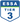•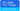•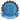•